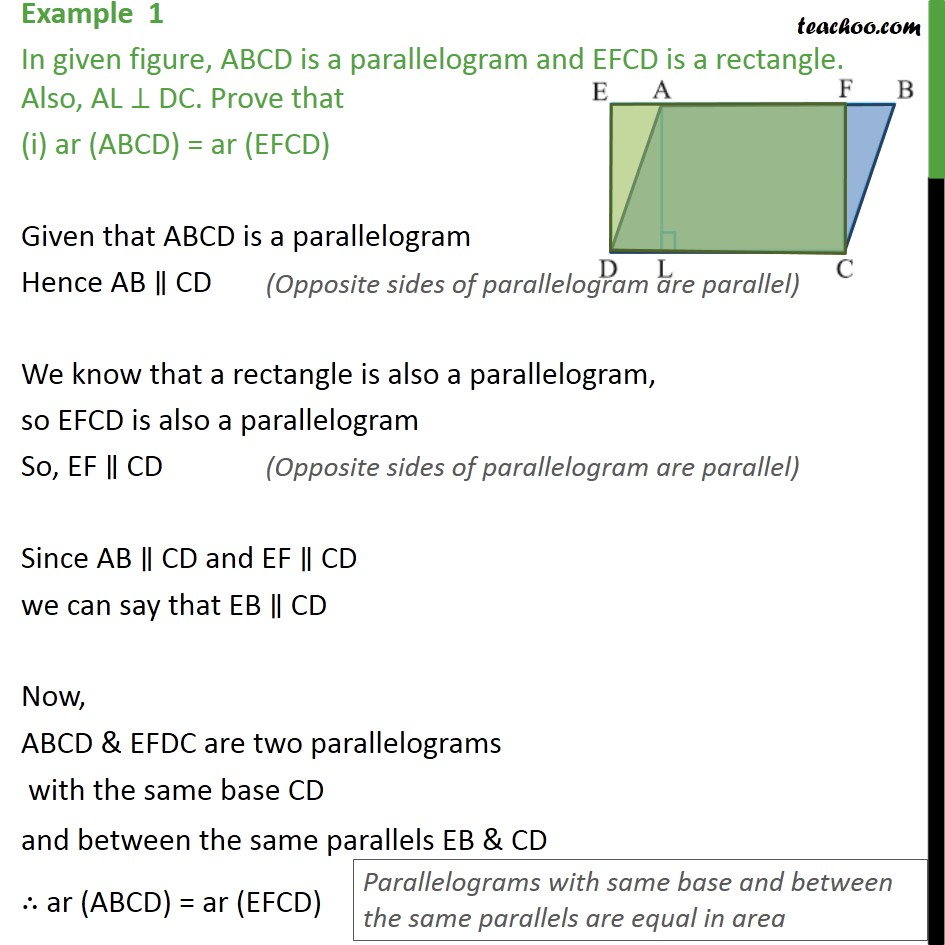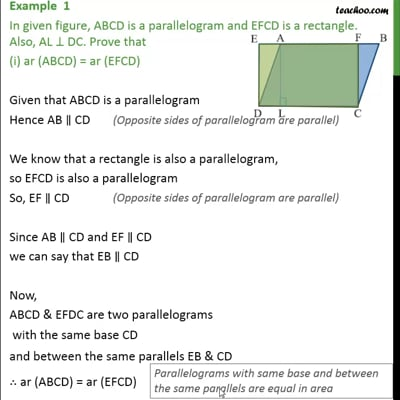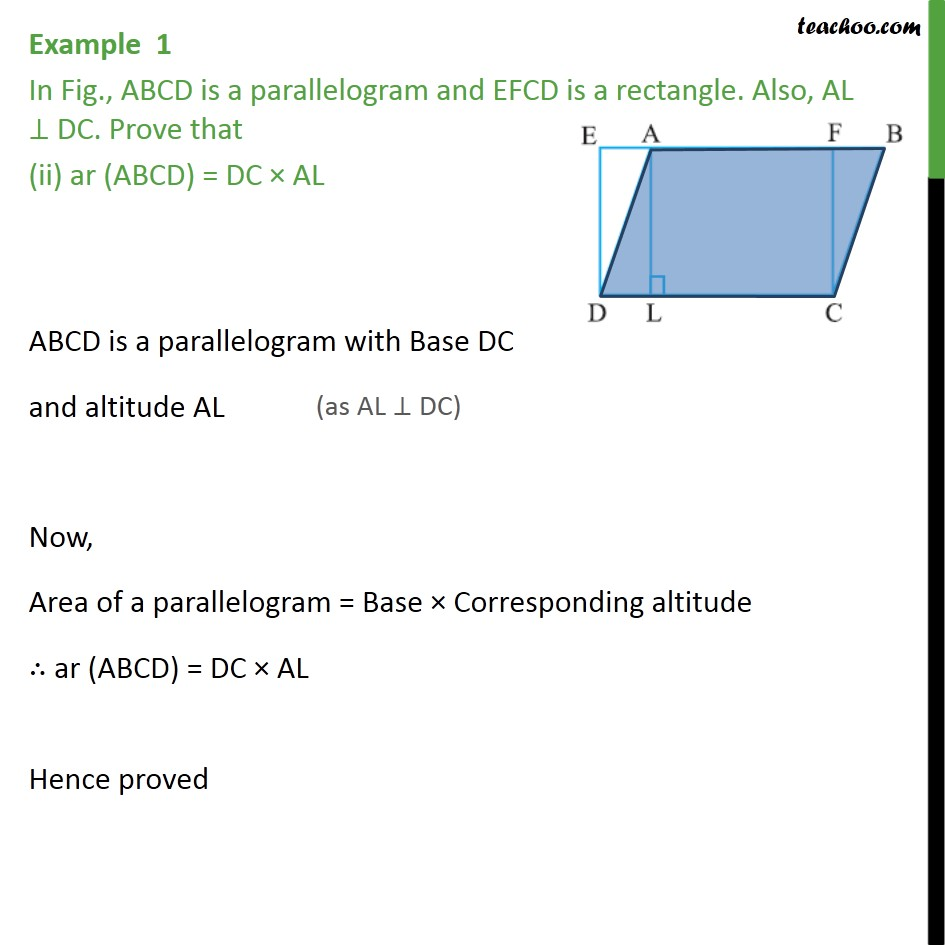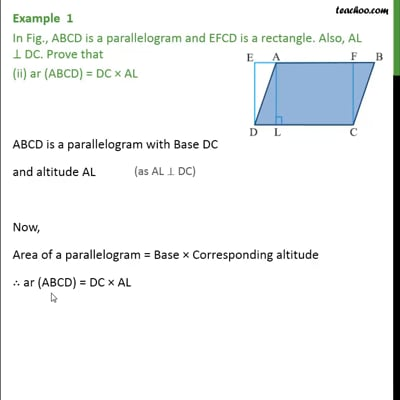Examples

Areas of Parallelograms and Triangles
Serial order wiseThis video is only available for Teachoo black usersThis video is only available for Teachoo black users

Learn in your speed, with individual attention - Teachoo Maths 1-on-1 Class

### Transcript

Example 1 In given figure, ABCD is a parallelogram and EFCD is a rectangle. Also, AL ⊥ DC. Prove that (i) ar (ABCD) = ar (EFCD) Given that ABCD is a parallelogram Hence AB ∥ CD We know that a rectangle is also a parallelogram, so EFCD is also a parallelogram So, EF ∥ CD Since AB ∥ CD and EF ∥ CD we can say that EB ∥ CD Now, ABCD & EFDC are two parallelograms with the same base CD and between the same parallels EB & CD ∴ ar (ABCD) = ar (EFCD) Example 1 In Fig., ABCD is a parallelogram and EFCD is a rectangle. Also, AL ⊥ DC. Prove that (ii) ar (ABCD) = DC × AL ABCD is a parallelogram with Base DC and altitude AL Now, Area of a parallelogram = Base × Corresponding altitude ∴ ar (ABCD) = DC × AL Hence proved